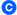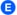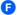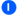# FC-200V

Close

• The colors may differ slightly from the original.

## FC-200V

Solar & Battery
Solar powered when light is sufficient, battery powered when light is insufficient.

## Features• Plastic keys
• Comes with new slide-on hard case
• Power supply:Solar cell and a single G13 type button battery (LR44)
• Approximate battery life:3 years (1hour of operation per day)
• Dimensions:12.2(H) × 80(W) × 161(D) mm
• Approximate weight:105g

### Direct mode key

A bank of mode keys provides you with one-touch access to the mode you need.1.Simple interest
Interest amount, principal and interest

2.Compound interest
Payment period, interest rate, deposit amount, future value

3.Investment Appraisal (cash flow)
Net present value method, internal rate of return method, payback period method, etc.

4.Amortization
Monthly payment, principal and interest to date

5.General and function
Virtually the same functions as a standard calculator.

6.Statistical and regression
Statistical calculations using input sample data.

7.Interest rate conversion
Nominal interest rate and effective interest rate conversion

8.Cost, selling price,or margin
Calculation of any of the above values after inputting the other two

9.Day or date calculations
Virtually the same as a standard calculator, with some variation in the input method

10.Depreciation
Straight-line method, declining balance method

11.Bond calculation
Purchase price, annual yield

12.Break-even point
Six modes for calculation of break-even point, etc.FC-200V FC-100V
Simple interestCompound interestInvestment Appraisal (cash flow)AmortizationInterest rate conversionCost,selling price,or marginDay or date calculationsDepreciationBond calculationBreak-even pointGeneral and FunctionStatistical and regression### Easy operation with parameters

A full-dot 4-line display provides easier scrolling between parameters and simplifies input, confirmation, and editing.### Calculate the result.The result appears immediately after you press the SOLVE key.

### Create shortcuts.Once you use a parameter value or setting in a calculation, you can assign it to a shortcut key for instant recall whenever you need it. This feature is great for repeat calculations.

## Specifications

• Non Programmable

•10 + 2 digits 10-digit mantissa + 2-digit exponential display.

•Solar & Battery Solar powered when light is sufficient, battery powered when light is insufficient.

•Key rollover Key operations are stored in a buffer, so nothing is lost even during high-speed input.

••Plastic keys Designed and engineered for easy operation.

• Basic Mathematical Functions Trigonometric, Exponential logarithmic, etc.

• Basic Statistics Standard statistics functions such as Mean, SUM, Standard Deviation, and Regression

• Financial Financial functions are built in, making financial calculations easy.

• Non Graphing

### Size of case / Total weight

• Dimensions (D × W × H) : 161×80×12.2mm
• Weight : 105g